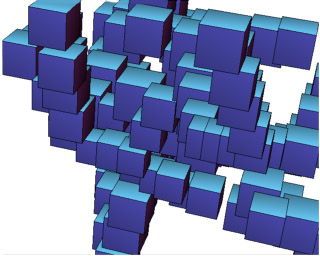# Mathematica and JavaView

JavaView provides an integrated viewer and geometry toolbox for Mathematica graphics. JavaView 4.0 understands most Mathematica graphics types and viewing options, and it is capable of providing user feedback such as pick information back into a Mathematica notebook session. For example, user events like picking with a mouse or dragging a vertex in a JavaView display may be caught by a Mathematica notebook and used as 2d or 3d input parameters.

Some application areas of the tight integration of Mathematica and JavaView:

•  View any Mathematica graphics and animation in a JavaView display
•  Receive pick information from JavaView into a Mathematica notebook
•  Interactive web pages with Mathematica graphics
•  Apply advanced algorithms of JavaView to extend Mathematica's rich set of algorithms
•  Export a Mathematica graphics into different file formats like Vrml, Stl ...
•  Full scripting of JavaView from a Mathematica notebook with J/Link
•  Create interactive web services with webMathematica and MSPJavaView[]

## Compare Mathematica Graphics in JavaView

The following links provide a complete comparison of the rendering quality in JavaView and Mathematica where each Mathematica graphics type and graphics options is rendered in a JavaView applet, and then compared to the original Mathematica picture on a separate page.

A quick overview provides the gallery with static images of Mathematica graphics and options rendered in JavaView.

#### Graphics Types and Options

The static images of the gallery appear as interactive applets in the subsequent web pages below. Each of the following pages contains a large number applets and is intended for developers only.

NOTE: If you encounter that some applets are not loaded or issue a memory warning, then ensure that your Java virtual machine has enough memory allocated.

## WebMathematica and JavaView

JavaView is a featured webMathematica site of Wolfram Research. Our JavaView enhanced webMathematica site includes many examples on the integration of webMathematica and JavaView More examples are given at the example section at Wolfram Research.

## Example: JavaView in a Notebook

Use JavaView within a Mathematica notebook to view graphics geometries. Start with the tutorial notebook Overview.nb which comes with the download package. The demonstration web pages above were generated from the notebooks Sample_JavaView.nb and Sample_JavaView_GraphicOptions.nb.

```<<JavaView`JLink` InstallJavaView[];```

`cube = Graphics3D[Cuboid[{0, 0, 0}, {5, 2, 1}]];`
`JavaView[cube];`Make graphics transparent, apply texture or invoke other visualization tools of JavaView directly from a Mathematica notebook:

```geom = JavaView[cube]; geom@showTransparency[True]; geom@setTransparency[0.4]; geom@update[geom]```A list of graphics objects may be animated in JavaView:

```cubeAnim = Table[Graphics3D[     Cuboid[10{Random[], Random[], Random[]}]], {20}]; JavaView[cubeAnim, Animatable->True];```Create a web page displaying a Mathematica graphics in a JavaView applet:

`WriteHtml[cubeAnim, "myAnim.html"];`